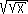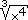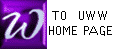THE ANTI-POWER RULE
(also called "POWER RULE for anti-derivatives")

Earlier we encountered the power rule used in finding derivatives:
 FUNCTION FORMAT: If f (x) = xn , then f '(x) = nxn-1 OPERATOR FORMAT: ddx xn = nxn-1

We now notice that we can translate the above rule for derivatives into the language of anti-derivatives, as stated below:

THE ANTI-POWER RULE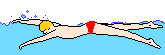FUNCTION FORMAT: If f (x) = xn , then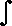f (x ) dx = 1  n+1 xn+1 + C
 OPERATOR FORMAT:xn dx = 1  n+1 xn+1 + C

We first encountered the table below as examples of the power rule for derivatives, and we then filled in the boxes of the right-most 2 columns. This earlier table is enhanced below to include exercizes solved using the power rule for anti-derivatives: the ANTI-POWER RULE. As we did earlier, the first 14 rows will be completed in class or in an on-line exercise, emphasizing the first column. The last 4 rows are left as problems on your take-home test. For several of these problems, rules for exponents will be helpful.f (x) dx =
FUNCTION
f (x) =
n = f '(x)
x2
x3
x10
x
1
1x

1x2

1x10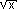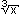1()2
xx.